# Speed Time Graph Worksheet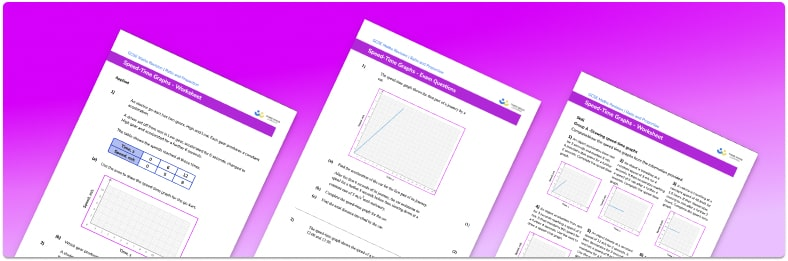• Section 1 of the speed time graph worksheet contains 18 skills-based speed time graph questions, in 3 groups to support differentiation
• Section 2 contains 4 applied speed time graph questions with a mix of worded problems and deeper problem solving questions
• Section 3 contains 4 foundation and higher level GCSE exam style practice questions including worded problems
• Answers and a mark scheme for all exam questions are provided
• Questions follow variation theory with plenty of opportunities for students to work independently at their own level
• All questions are created by fully qualified expert secondary maths teachers
• Suitable for GCSE maths revision for AQA, OCR and Edexcel exam boards

• This field is for validation purposes and should be left unchanged.

You can unsubscribe at any time (each email we send will contain an easy way to unsubscribe). To find out more about how we use your data, see our privacy policy.

### Speed time graph at a glance

A speed-time graph shows how the speed of an object varies over time. On any speed-time graph or velocity-time graph, a positive gradient represents acceleration, a horizontal line represents constant speed and a negative gradient represents deceleration.

We can draw a speed-time graph if we are given key information about a journey. We may need to use the formula Speed = Distance / Time to calculate unknown values.

On a speed-time graph, the acceleration at any time is equal to the gradient of the line at that time. Constant acceleration is therefore shown by a straight line. Average acceleration between two times can be found by drawing a line between the speed at those two times and finding the gradient of the line.

The average speed of an object, e.g. a cyclist, over the course of an entire journey can be calculated by dividing the total distance travelled by the total time taken.

You may also see speed-time graphs in GCSE physics.

Looking forward, students can then progress to additional rate of change worksheets and other ratio and proportion worksheets, for example a ratio worksheet or a simplifying and equivalent ratios worksheetFor more teaching and learning support on Ratio and Proportion our GCSE maths lessons provide step by step support for all GCSE maths concepts.

When you have students who require more intensive support our one to one GCSE maths revision programme will match them with the most appropriate tutor. This way we can provide individual students with personalised programmes of study while you continue to teach the rest of your class as a whole group.

Our maths interventions are currently only available for GCSE students, and are not suitable for A level students.

## Related worksheets

Rate Of Change Worksheet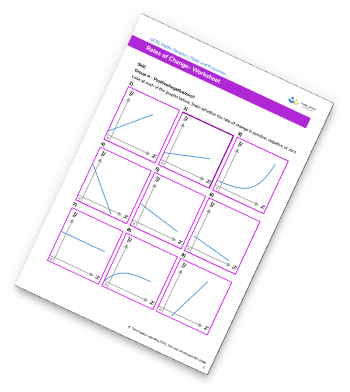Ratio To Fraction Worksheet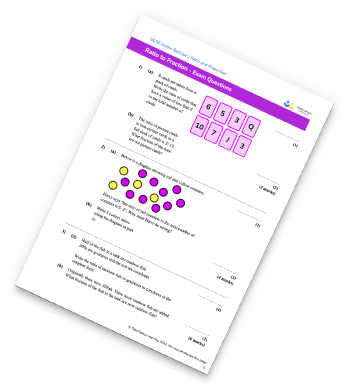Simplifying Ratios Worksheet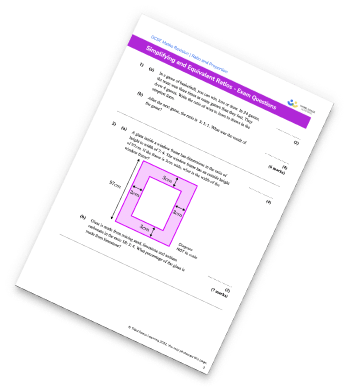Ratio Worksheet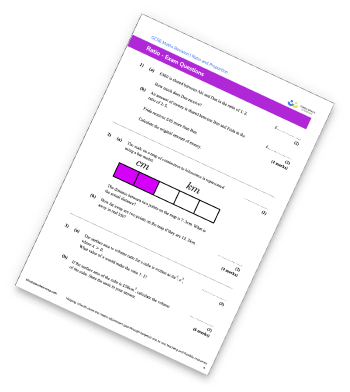## Do you have KS4 students who need more focused attention to succeed at GCSE?There will be students in your class who require individual attention to help them succeed in their maths GCSEs. In a class of 30, it’s not always easy to provide.

Help your students feel confident with exam-style questions and the strategies they’ll need to answer them correctly with our dedicated GCSE maths revision programme.

Lessons are selected to provide support where each student needs it most, and specially-trained GCSE maths tutors adapt the pitch and pace of each lesson. This ensures a personalised revision programme that raises grades and boosts confidence.

Find out more